Updated 26/09/2023
In force

Version from: 28/06/2023
Amendments
There is currently no level 2 legal act based on or specifying Article 280e.
Search within this legal act

### Article 280e - Commodity risk category add-on

Article 280e

1.

For the purposes of Article 278, institutions shall calculate the commodity risk category add-on for a given netting set as follows:where:

 j = the index that denotes the commodity hedging sets established in accordance with point (e) of Article 277a(1) and with Article 277a(2) for the netting set; and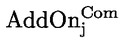= the commodity risk category add-on for hedging set j calculated in accordance with paragraph 4.
2.
For the purpose of calculating the add-on for a commodity hedging set of a given netting set in accordance with paragraph 4, institutions shall establish the relevant commodity reference types of each hedging set. Commodity derivative transactions shall be assigned to the same commodity reference type only where the underlying commodity instrument of those transactions has the same nature, irrespective of the delivery location and quality of the commodity instrument.
3.
By way of derogation from paragraph 2, competent authorities may require an institution which is significantly exposed to the basis risk of different positions sharing the same nature as referred to in paragraph 2 to establish the commodity reference types for those positions using more characteristics than just the nature of the underlying commodity instrument. In such a situation, commodity derivative transactions shall be assigned the same commodity reference type only where they share those characteristics.
4.

Institutions shall calculate the commodity risk category add-on for hedging set j as follows: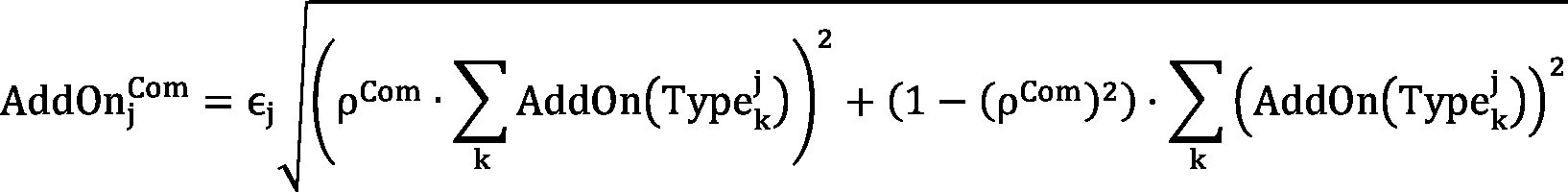where:= the commodity risk category add-on for hedging set j;
 єj = the hedging set supervisory factor coefficient of hedging set j determined in accordance with Article 280;
 ρCom = the correlation factor of the commodity risk category with a value equal to 40 %;
 k = the index that denotes the commodity reference types of the netting set established in accordance with paragraph 2; and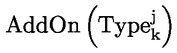= the add-on for the commodity reference type k calculated in accordance with paragraph 5.
5.

Institutions shall calculate the add-on for the commodity reference type k as follows: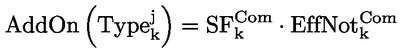where:= the add-on for the commodity reference type k;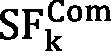= the supervisory factor applicable to the commodity reference type k; where the commodity reference type k corresponds to transactions allocated to the hedging set referred to in point (e) of Article 277a(1), excluding transactions concerning electricity,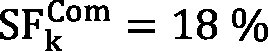; for transactions concerning electricity,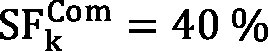; and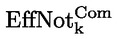=

the effective notional amount of the commodity reference type k calculated as follows: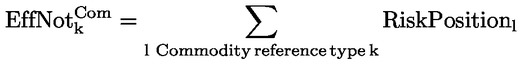where:

 l = the index that denotes the risk position.